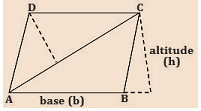# Area of a Parallelogram Formula

Parallelogram is a geometrical figure that has four sides formed by two pairs of parallel lines. Opposite sides are equal in length and opposite angles are equal in measure. The base and height of a parallelogram must be perpendicular. However, the lateral sides of a parallelogram are not perpendicular to the base. Thus, a dotted line is drawn to represent the height. The area of a parallelogram is also equal to the magnitude of the vector cross product of two adjacent sides. The area of a triangle created by one of its diagonals. A picture of a leaning box or rectangle is the best example of a parallelogram.

## Area of a ParallelogramArea = b $\times$ h
Where,
Where, b is the length of any base and h is the corresponding altitude or height

Solved Examples
Question 1: Find the area of a parallelogram whose breadth is 8 cm and height is 11 cm ?
Solution:
Given,
b = 8 cm
h = 11 cm
Area of a parallelogram
= b $\times$ h
= 8 $\times$ 11 cm2
= 88 cm2
Question 2: The base of the parallelogram is thrice its height. If the area is 192 $cm^{2}$, find the base and height.
Solution:
Let the height of the parallelogram = h cm
then, the base of the parallelogram = 3h cm
Area of the parallelogram = 192 $cm^{2}$
Area of parallelogram = base × height
Therefore, 192 = 3h × h
3 $\times$ $h^{2}$ = 192
$h^{2}$ = 64
h = 8 cm

Hence the height of the parallelogram is 8 cm and breath is
3 $\times$ h
= 3 $\times$ 8
= 24 cm